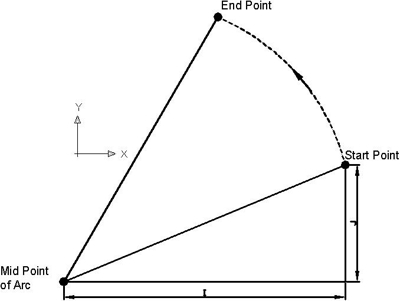# Circular Interpolation Concepts & Programming Part 4 (Unknown R)

The 4th part of Circular Interpolation Concepts & Programming series explains how cnc program for an arc can be made if arc Radius (R) is not directly given.

## Program an Arc only Arc Center is given

To program an arc when only the arc center is given (the radius is unknown) use the address letters I and J

• I – relates to the address X and is the incremental value and direction (+/-) from the start point of the arc in the X axis to the arc centre.
• J – relates to the address Y and is the incremental value and direction (+/-) from the start point of the arc in the Y axis to the arc centre.Program an Arc only Arc Center is given

In this figure the procedure is very clear to calculate I and J values. From the point where arc is started always look to wards arc midpoint.  If the arc is less than a quadrant than you must find out the both I and J values.

`G03 X (End Point) Y(End Point) I J`

For CNC Lathe Machinists
Fanuc Circular Interpolation G02 G Code Example